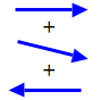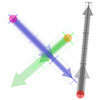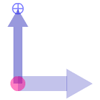#### You may also like### Arrow Arithmetic 1

The first part of an investigation into how to represent numbers using geometric transformations that ultimately leads us to discover numbers not on the number line.### Arrow Arithmetic 3

How can you use twizzles to multiply and divide?### Twizzle Arithmetic

Arrow arithmetic, but with a twist.

# Arrow Arithmetic 2

##### Age 14 to 16 Challenge Level:

This problem follows on from Arrow Arithmetic 1

We're trying to find a way of drawing numbers that will also help us draw not just the number, but also make sense of number operations such as addition and multplication.

In Arrow Arithmetic 1, we tried using a single arrow to represent a number. This has some features we desire since we can draw long arrows to represent big numbers and short arrows for small numbers. The direction of the arrow should allow us to distinguish positive from negative numbers.

But as we saw, there is a problem with using a single arrow, which is captured in this question: 'How long does an arrow need to be to represent the number 1, and which way should it point? '

Once we answer this question by choosing a unit arrow, it becomes very easy to represent other numbers with arrows. 2 is an arrow twice as long as the unit arrow, pointing the same way. -3 is an arrow three times as long as the unit arrow, pointing in the opposite direction.

These thoughts led me to the idea of using two arrows to picture a number. I'm going to call this pair of arrows a twizzle. The thicker arrow in the twizzle is the unit arrow - which we can choose to be any length we like and to point in any direction. The other arrow in the twizzle is the number arrow. It determines the value of the twizzle by comparison to the unit arrow. Play with this animation until you understand how it works, and how the twizzle respresents a number. Check with the hints to ensure you have discovered all the ways to manipulate twizzles.

Full Screen Version
If you can see this message Flash may not be working in your browser
Please see http://nrich.maths.org/techhelp/#flash to enable it.

In the next animation I am combining the blue and green twizzles together to make a grey twizzle.

I now have two questions for you:
How is the value of the grey twizzle related to the value of the blue and green twizzles?
How would you construct the grey twizzle from the blue and green twizzles using rotations, translations, and enlargements?

Full Screen Version
If you can see this message Flash may not be working in your browser
Please see http://nrich.maths.org/techhelp/#flash to enable it.

How would the value of a twizzle change if you rotated its number arrow through 180 degrees?
Explain how you could use this fact to construct twizzle subtraction.

Full Screen Version
If you can see this message Flash may not be working in your browser
Please see http://nrich.maths.org/techhelp/#flash to enable it.